﻿ 基于SVM的状态反馈故障诊断技术 State Feedback Fault Diagnosis Technology Based on SVM

Dynamical Systems and Control
Vol.07 No.01(2018), Article ID:23181,16 pages
10.12677/DSC.2018.71002

State Feedback Fault Diagnosis Technology Based on SVM

Zhiming Fang1, Fuzhong Wang2, Bo Yao1

1College of Mathematics and System Science, Shenyang Normal University, Shenyang Liaoning

2Department of Basic Education, Shenyang Institute of Engineering, Shenyang Liaoning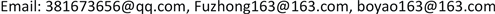Received: Dec. 2nd, 2017; accepted: Dec. 20th, 2017; published: Dec. 27th, 2017ABSTRACT

In this paper, SVM is used to classify the poles of the system, and the poles generated by the state feedback system without faults and faults are distinguished. And the grid search method is used to find the optimal parameters, and the pole classification method is modeled. In order to realize the real-time monitoring of system pole change, a new method to estimate the system poles by system status is presented. On the basis of the fault diagnosis model, a reliable controller is designed for the corresponding faults. Finally, an example is given to verify the correctness of the pole estimation method, the accuracy of the fault diagnosis and the effectiveness of the reliable controller.

Keywords:Pole Estimation Method, Pole Classification Model, SVM, Fault Diagnosis, State Feedback

1沈阳师范大学数学与系统科学学院，辽宁 沈阳

2沈阳工程学院基础教学部，辽宁 沈阳Copyright © 2018 by authors and Hans Publishers Inc.1. 引言

SVM是近年来兴起的一种基于统计学习理论的机器学习算法，以结构风险最小化为原则来训练和建立模型，其算法本质上是一个凸二次优化问题，能够保证找到的极值解就是全局最优解   。SVM善于解决小样本、非线性和高维数问题，具有结构简单、泛化能力优秀等特点。有些学者将统计学习理论应用到系统辨识和故障诊断领域   ，其中对于支持向量机应用的研究也有了一定的发展    。一些学者将支持向量机应用到了故障分类中，文献  和文献  分别给出了一种基于最小二乘支持向量机的故障分类方案。文献  和  将SVM应用到了模糊控制中，并且文献  对SVM的算法进行了改进，首次提出用一个新的回归来解决最小二乘支持向量机中的问题，使其学习速度得到了提升。

2. 基于LIB-SVM的控制系统中极点配置问题的故障诊断方法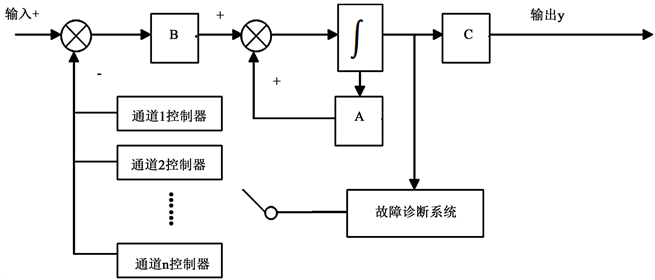Figure 1. System principle of fault diagnosis for state feedback system based on LIB-SVM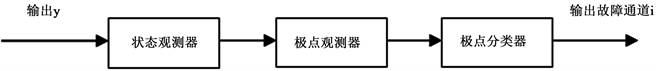Figure 2. Structure of fault diagnosis system

2.1. 状态观测器设计

$\left\{\begin{array}{l}\stackrel{˙}{x}=Ax+Bu\\ y=Cx\end{array}$ (1)

$\stackrel{^}{y}\left(t\right)-y\left(t\right)=C\left[\stackrel{^}{x}\left(t\right)-x\left(t\right)\right]$ (2)

$\stackrel{˙}{\stackrel{^}{x}}=\left(A-EC\right)\stackrel{^}{x}+Bu+E$ (3)

2.2. 极点观测器设计

2.2.1. 问题描述

$\stackrel{˙}{x}=Ax\left(t\right),\text{\hspace{0.17em}}\text{\hspace{0.17em}}x\left(t\right)={\left[\begin{array}{ccc}{x}_{1}\left(t\right)& \cdots & {x}_{n}\left(t\right)\end{array}\right]}^{\text{T}},\text{\hspace{0.17em}}\text{\hspace{0.17em}}\stackrel{˙}{x}\left(t\right)={\left[\begin{array}{ccc}{\stackrel{˙}{x}}_{1}\left(t\right)& \cdots & {\stackrel{˙}{x}}_{n}\left(t\right)\end{array}\right]}^{\text{T}}$ (4)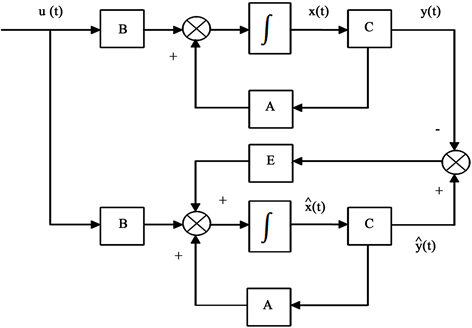Figure 3. Structure diagram of full dimensional state observer

${{x}^{\prime }}_{0}=x\left({t}_{0}\right),\text{\hspace{0.17em}}{{x}^{\prime }}_{1}=x\left({t}_{0}+\Delta t\right),\text{\hspace{0.17em}}{{x}^{\prime }}_{2}=x\left({t}_{0}+2\Delta t\right),\cdots ,{{x}^{\prime }}_{n}=x\left({t}_{0}+n\Delta t\right)$

2.2.2主要结果

$x=x\left(t\right)$ ，则 $\text{d}x\left(t\right)=\stackrel{˙}{x}\left(t\right)\text{d}t$ ，可以得到近似计算公式

$\Delta x=\stackrel{˙}{x}\left(t\right)\Delta t$ (5)

$\Delta {x}^{i}=\stackrel{˙}{x}\left({t}_{0}+i\Delta t\right)-\stackrel{˙}{x}\left({t}_{0}+\left(i-1\right)\Delta t\right)$ (6)

$\Delta {x}^{1}={x}^{1}-{x}^{0}=x\left({t}_{0}+\Delta t\right)-x\left(t0\right)$

$\Delta {x}^{2}={x}^{2}-{x}^{1}=x\left({t}_{0}+2\Delta t\right)-x\left({t}_{0}+\Delta t\right)$

$\cdots$

$\Delta {x}^{n}={x}^{n}-{x}^{n-1}=x\left({t}_{0}+n\Delta t\right)-x\left({t}_{0}+\left(n-1\right)\Delta t\right)$

$\Delta {x}^{i}=\stackrel{˙}{x}\left({t}_{0}+\left(i-1\right)\Delta t\right)\Delta t$

$\Delta {x}^{i}=Ax\left({t}_{0}+\left(i-1\right)\Delta t\right)\Delta t$ (7)

$\left[\begin{array}{cccc}\Delta {x}^{1}& \Delta {x}^{2}& \cdots & \Delta {x}^{n}\end{array}\right]=A\left[\begin{array}{cccc}x\left({t}_{0}\right)& x\left({t}_{0}+\Delta t\right)& \cdots & x\left({t}_{0}+n\Delta t\right)\end{array}\right]\Delta t$

$\Delta X=\left[\begin{array}{cccc}\Delta {x}^{1}& \Delta {x}^{2}& \cdots & \Delta {x}^{n}\end{array}\right]$

$X=\left[\begin{array}{cccc}x\left({t}_{0}\right)& x\left({t}_{0}+\Delta t\right)& \cdots & x\left({t}_{0}+n\Delta t\right)\end{array}\right]\Delta t$

$X$ 可逆，则得到系统矩阵的估算矩阵 $\Delta X\approx AX$

$A\approx \Delta X{X}^{-1}$ (8)

2.3. 极点分类器设计

2.3.1. 极点分类器预测模型设计

LIBSVM是中国台湾大学林智仁教授等开发设计的软件对SVM所涉及的参数调节相对比较少，并且提供了很多的默认参数，利用这些默认参数可以解决很多问题。支持向量C-SVC基本原理如下：

$\underset{w,b,\xi }{\mathrm{min}}\frac{1}{2}{w}^{\text{T}}w+C\sum _{i=1}^{l}\text{ }\text{ }{\xi }_{i}$

$\text{s}\text{.t}\text{.}\text{\hspace{0.17em}}\text{\hspace{0.17em}}{y}_{i}\left({w}^{\text{T}}\varnothing \left({x}_{i}\right)+b\right)\ge 1-{\xi }_{i}$

${\xi }_{i}\ge 0,\text{\hspace{0.17em}}i=1,\cdots ,l$

$\underset{\alpha }{\mathrm{min}}\frac{1}{2}{\alpha }^{\text{T}}Q\alpha +{e}^{\text{T}}\alpha$

$\text{s}\text{.t}.\text{\hspace{0.17em}}\text{\hspace{0.17em}}{y}^{\text{T}}\alpha =0$

$0\le {\alpha }_{i}\le C,\text{\hspace{0.17em}}i=1,\cdots ,l$

$\mathrm{sgn}\left(\sum _{i=1}^{l}\text{ }\text{ }{y}_{i}{\alpha }_{i}K\left({x}_{i},x\right)+b\right)$

1) 收集数据：收集故障部件n条通道分别出现故障时的极点数据，对数据进行标准化处理；

2) 划分训练集与测试集：分别选取n条通道故障时极点数据的一半作为训练集，另一半作为测试集；

3) 将Accuracy作为评价标准，首先建立粗糙预测模型；

4) 参数寻优：采用网格搜寻法，选取最佳的参数c和g，建立模型；

5) 提取模型：提取使Accuracy达到最高的参数c和g，作为预测模型的最终参数建立极点分类模型。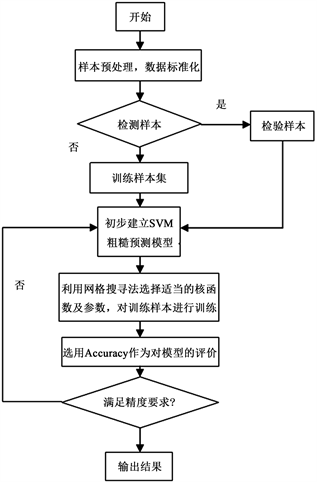Figure 4. Modeling process

2.3.2. 极点分类处理

$D=\left\{s\in C:L+sM+\overline{s}{M}^{\text{T}}<0\right\}$

${f}_{D}\left(z\right)=L+sM+\overline{s}{M}^{\text{T}}$

2.4. 单通道控制器的设计

$\left\{\begin{array}{l}\stackrel{˙}{x}\left(t\right)=Ax\left(t\right)+Bu\left(t\right)\\ y\left(t\right)=Cx\left(t\right)\end{array}$ (9)

${u}^{f}\left(t\right)={F}_{a}u\left(t\right)$ (10)

${M}_{D}\left(A,X\right)<0$

${M}_{D}\left(A,X\right)=L\otimes X+M\otimes \left(AX\right)+{M}^{\text{T}}\otimes {\left(AX\right)}^{\text{T}}$

$\left[\begin{array}{cc}-rX& AX+qX\\ X{A}^{\text{T}}+qX& -rX\end{array}\right]<0$

${\phi }_{ai2}=\text{diag}\left(1,\cdots ,1,{\overline{f}}_{ai},1,\cdots ,1\right),\text{\hspace{0.17em}}i=1,2,\cdots ,p$

3. 主要结果

3.1. 线性系统圆形区域极点配置状态反馈控制器设计

$u\left(t\right)=Kx\left(t\right)$ (11)

$\stackrel{˙}{x}\left(t\right)=\left(A+BK\right)x\left(t\right)$ (12)

$\left[\begin{array}{cc}-rX& \left(A+qI\right)X+BY\\ X{\left(A+qI\right)}^{\text{T}}+{Y}^{\text{T}}{B}^{\text{T}}& -rX\end{array}\right]<0$

$\left[\begin{array}{cc}-rX& AX+qX\\ X{A}^{\text{T}}+qX& -rX\end{array}\right]<0$ (13)

$\left[\begin{array}{cc}-rX& \left(A+BK\right)X+qX\\ X{\left(A+BK\right)}^{\text{T}}+qX& -rX\end{array}\right]<0$ (14)

$\left[\begin{array}{cc}-rX& \left(A+BY{X}^{-1}\right)X+qX\\ X{\left(A+BY{X}^{-1}\right)}^{\text{T}}+qX& -rX\end{array}\right]<0$

$\left[\begin{array}{cc}-rX& \left(A+qI\right)X+BY\\ X{\left(A+qI\right)}^{\text{T}}+{Y}^{\text{T}}{B}^{\text{T}}& -rX\end{array}\right]<0$

3.2. 线性系统执行器故障圆形区域极点配置状态反馈控制器设计

$\left\{\begin{array}{l}\stackrel{˙}{x}\left(t\right)=Ax\left(t\right)+B{F}_{a}u\left(t\right)\\ y\left(t\right)=Cx\left( t \right)\end{array}$

${u}^{f}\left(t\right)={F}_{a}Kx\left( t \right)$

$\stackrel{˙}{x}\left(t\right)=\left(A+B{F}_{a}K\right)x\left(t\right)$ (15)

$\left[\begin{array}{cc}-rX& \left(AX+B{\phi }_{ai}Y\right)+qX\\ \left(X{A}^{\text{T}}+{Y}^{\text{T}}{\phi }_{ai}{B}^{\text{T}}\right)+qX& -rX\end{array}\right]<0$

$\left[\begin{array}{cc}-rX& AX+qX\\ X{A}^{\text{T}}+qX& -rX\end{array}\right]<0$ (16)

$\left[\begin{array}{cc}-rX& \left(A+B{F}_{a}K\right)X+qX\\ X{\left(A+B{F}_{a}K\right)}^{\text{T}}+qX& -rX\end{array}\right]<0$ (17)

$\left[\begin{array}{cc}-rX& \left(AX+B{F}_{a}Y\right)+qX\\ \left(X{A}^{\text{T}}+{Y}^{\text{T}}{F}_{a}{B}^{\text{T}}\right)+qX& -rX\end{array}\right]<0$ (18)

$\left[\begin{array}{cc}-rX& \left(AX+B{\phi }_{ai}Y\right)+qX\\ \left(X{A}^{\text{T}}+{Y}^{\text{T}}{\phi }_{ai}{B}^{\text{T}}\right)+qX& -rX\end{array}\right]<0$

4. 系统仿真

$\left[\begin{array}{c}{\stackrel{˙}{x}}_{1}\\ {\stackrel{˙}{x}}_{2}\\ {\stackrel{˙}{x}}_{3}\end{array}\right]=\left[\begin{array}{ccc}5& 1& 0\\ 0& 2& 0\\ 1& 1& -2\end{array}\right]\left[\begin{array}{c}{x}_{1}\\ {x}_{2}\\ {x}_{3}\end{array}\right]+\left[\begin{array}{cc}1& 0\\ 1& -1\\ 0& -1\end{array}\right]u$

$y=\left[\begin{array}{ccc}1& 1& 0\\ 1& 0& 1\end{array}\right]\left[\begin{array}{c}{x}_{1}\\ {x}_{2}\\ {x}_{3}\end{array}\right]$

$\left[\begin{array}{c}{\stackrel{˙}{x}}_{1}\\ {\stackrel{˙}{x}}_{2}\\ {\stackrel{˙}{x}}_{3}\end{array}\right]=\left[\begin{array}{ccc}-2.0320& 0.0135& -0.0233\\ -1.4920& -1.0034& 0.0058\\ -4.5399& 3.0169& -2.0291\end{array}\right]\left[\begin{array}{c}{x}_{1}\\ {x}_{2}\\ {x}_{3}\end{array}\right]$

$\left[\begin{array}{c}{u}_{1}\\ {u}_{2}\end{array}\right]=\left[\begin{array}{ccc}-7.0320& -0.9865& -0.0233\\ -5.5399& 2.0169& -0.0291\end{array}\right]\left[\begin{array}{c}{x}_{1}\\ {x}_{2}\\ {x}_{3}\end{array}\right]$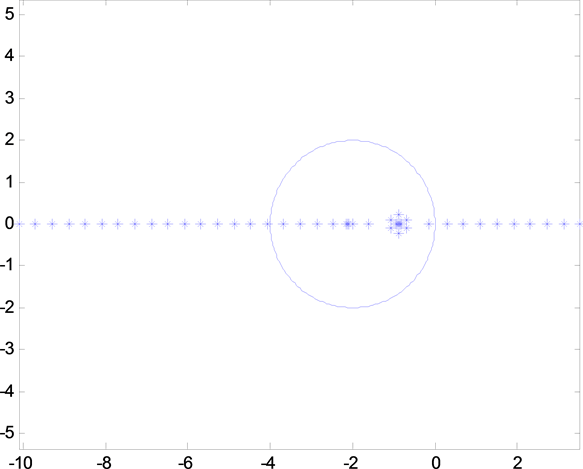Figure 5. Pole position diagram when channel 1 fails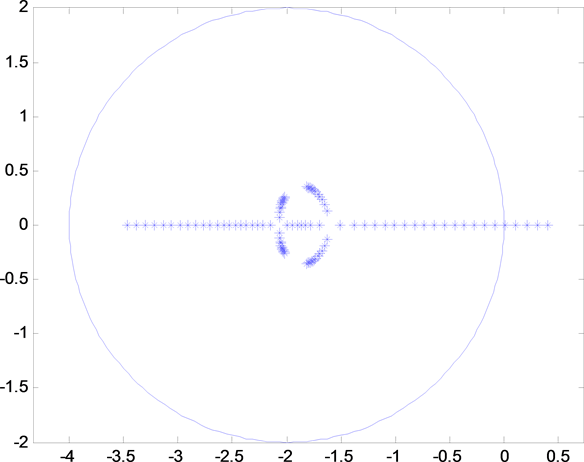Figure 6. Pole position diagram when channel 2 fails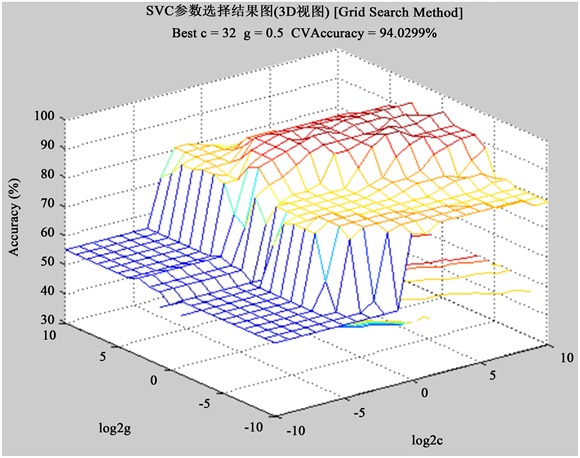Figure 7. Parameter selection 3D view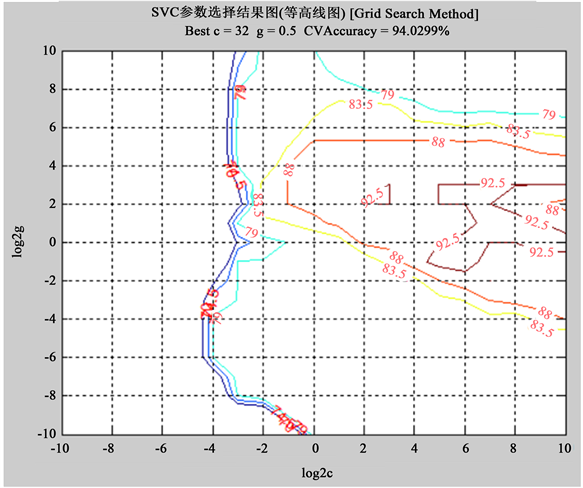Figure 8. Parameter selection contour view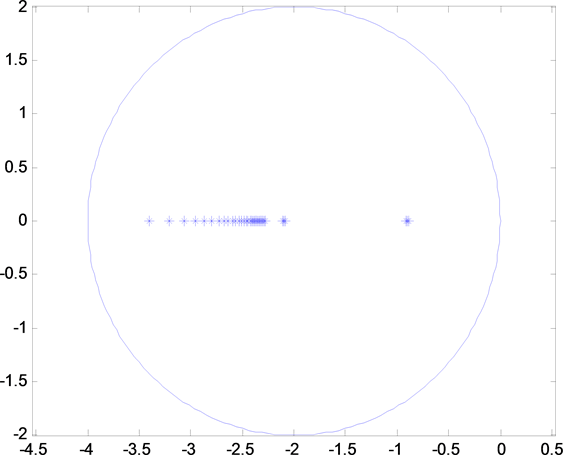Figure 9. Pole assignment diagram for channel 1 Failure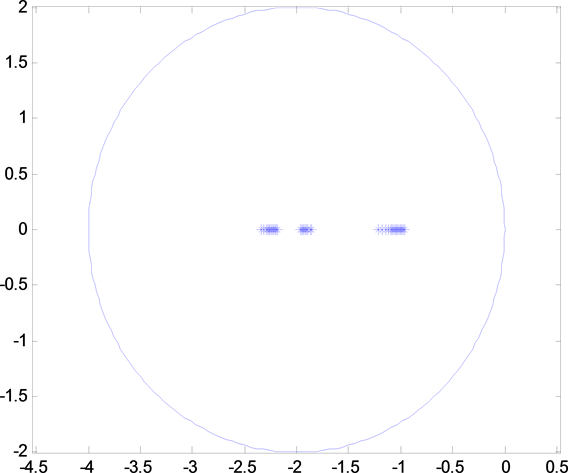Figure 10. Pole assignment diagram for channel 2 failure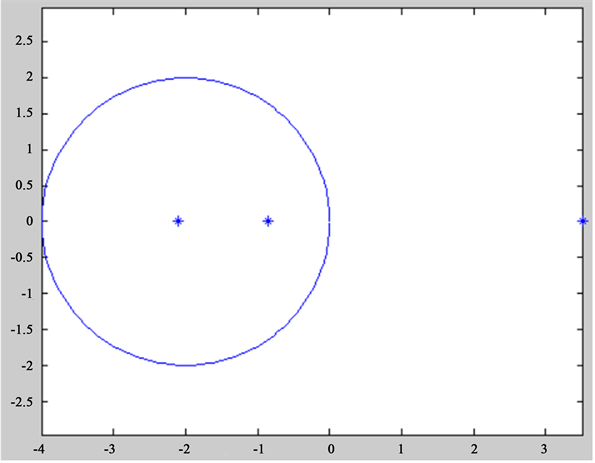Figure 11. Position diagram of fault pole

$x\left(t\right)={\text{e}}^{At}x\left( 0 \right)$

${{x}^{\prime }}_{1}={\left[\begin{array}{ccc}1.4299& 1.3774& 0.6426\end{array}\right]}^{\prime }$

${{x}^{\prime }}_{2}={\left[\begin{array}{ccc}1.4818& 1.4231& 0.6061\end{array}\right]}^{\prime }$

${{x}^{\prime }}_{3}={\left[\begin{array}{ccc}1.5355& 1.4705& 0.5693\end{array}\right]}^{\prime }$

${{x}^{\prime }}_{4}={\left[\begin{array}{ccc}1.5912& 1.5195& 0.5323\end{array}\right]}^{\prime }$

$\Delta x=\left[\begin{array}{ccc}{x}_{2}-{x}_{1}& {x}_{3}-{x}_{2}& {x}_{4}-{x}_{3}\end{array}\right]$

$X=\left[\begin{array}{ccc}{x}_{1}& {x}_{2}& {x}_{3}\end{array}\right]\ast \Delta t$

$X$ 可逆，根据式子(8) $A=\Delta x\ast {X}^{-1}$ ，求出：

${\lambda }^{\prime }=\left\{-2.0882,-0.8589,3.5840\right\}$

5. 结论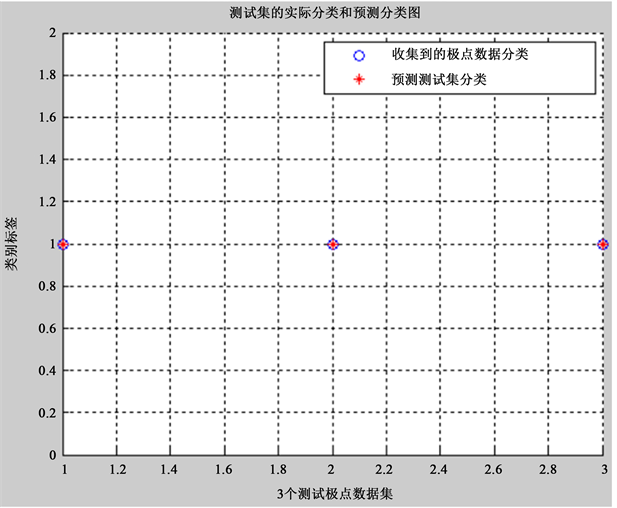Figure 12. Pole classification and prediction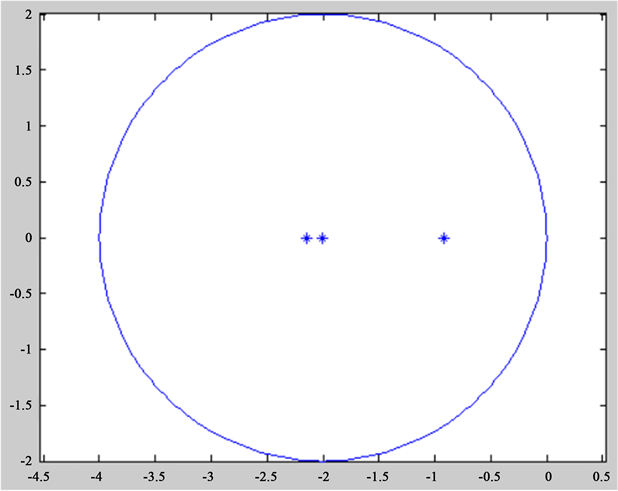Figure 13. Pole assignment diagram

State Feedback Fault Diagnosis Technology Based on SVM[J]. 动力系统与控制, 2018, 07(01): 11-26. http://dx.doi.org/10.12677/DSC.2018.71002

1. 1. Vapnik, V.N. (1998) Statistical Learning Theory. John Wiley, New York.

2. 2. Vapnik, V.N. (1995) The Nature of Statistical Learning Theory. Springer-Verlag, New York.
https://doi.org/10.1007/978-1-4757-2440-0

3. 3. Yen, C.-W., Young, C.-N. and Nagurka, M.L. (2005) A Training Sample Sequence Planning Method for Pattern Recognition Problems. Automatica, 41, 575-581.
https://doi.org/10.1016/j.automatica.2004.10.012

4. 4. 杨娟, 陆阳, 黄镇谨. 基于二进神经网络0/1分布系统可靠性分析[J]. 自动化学报, 2014, 40(7): 1472-1480.

5. 5. Ong, C.J., Sui, D. and Gilbert, E.G. (2006) Enlarging the Terminal Region of Nonlinear Model Predictive Control Using the Support Vector Machine Method. Automatica, 42, 1011-1016.
https://doi.org/10.1016/j.automatica.2006.02.023

6. 6. Laurain, V., Tóth, R., Piga, D. and Zheng, W.X. (2015) An Instrumental Least Squares Support Vector Machine for Nonlinear System Identification. Automatica, 54, 340-347.
https://doi.org/10.1016/j.automatica.2015.02.017

7. 7. Ong, C.J., Keerthi, S.S., Gilbert, E.G. and Zhang, Z.H. (2004) Stability Regions for Constrained Nonlinear Systems and Their Functional Characterization via Support-Vector-Machine Learning. Automatica, 40, 1955-1964.
https://doi.org/10.1016/j.automatica.2004.06.005

8. 8. Dubey, H.C., Tiwari, A.K., Nandita and Kishor, N. (2012) A Novel Fault Classification Scheme Based on Least Square SVM. 2012 Students Conference on Engineering and Systems (SCES), IEEE, 1-5.

9. 9. Dash, P.K., Samantaray, S.R. and Panda, G. (2007) Fault Classification and Section Identification of an Advanced Series-Compensated Transmission Line Using Support Vector Machine. IEEE Transac-tions on Power Delivery, 22, 67-73.

10. 10. Chiang, J.-H. and Hao, P.-Y. (2004) Support Vector Learning Mechanism for Fuzzy Rule-Based Modeling: A New Approach. IEEE Transactions on Fuzzy Systems, 12, 1-12.
https://doi.org/10.1109/TFUZZ.2003.817839

11. 11. Zhao, W.Q., Zhang, J.J. and Li, K. (2015) An Efficient LS-SVM-Based Method for Fuzzy System Construction. IEEE Transactions on Fuzzy Systems, 23, 627-643.
https://doi.org/10.1109/TFUZZ.2014.2321594

12. 12. 姚波, 王福中. 基于LMI可靠跟踪控制器设计[J]. 自动化学报, 2001(5): 615-619.

13. 13. 王福中, 姚波. 基控制于LMI双故障动态输出反馈完整性[J]. 控制理论与应用, 2006(5): 976-980.

14. 14. Basher, H.A. (2013) An LMI Approach for Observer Based Model Reference Control. 1-5.

15. 15. Juloski, A.L., Heemels, W.P.M.H., Boers, Y., et al. (2003) Two Approaches to State Estimation for a Class of Piecewise Affine Systems. Proceedings of the IEEE Conference on Decision and Control, 1, 143-148.

16. 16. 郭胜辉, 朱芳来. 基于区间观测器的执行器故障检测[J]. 控制与决策, 2016, 31(6): 1118-1122.

17. 17. 朱芳来, 岑峰, 董学平. 一种基于全维和降维观测器的故障检测和重构方法[J]. 控制与决策, 2011, 26(2): 258-262.

18. 18. 张嗣瀛, 高立群. 现代控制理论[M]. 北京: 清华大学出版社, 2006: 219-220.